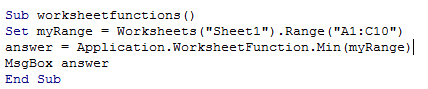## Vba worksheet functions in Excel

In the previous chapters, we saw a number of built-in functions of VBA which performs some specific functions.

Some of them are CStr(), Cint(), InStr(),Abs(), Exp() and so on, that performs some action.

But there are some functions which are much needed in VBA program, but is not available as built-in function.

Some of such functions are SumIf(),SumIfs(),CountIf(),CountIfs(),VLookUp(),HLookUp() and so on.

These functions are often need to be used but is not available with VBA.

Worksheetfunctions are used in this scenario.As the name implies, this function gives access to all the functions used

in Excel sheet which are not readily available in VBA.

For example, However complex a VBA application maybe, there might arise a situation where one want to search

for a value using VLookUp().In such case, Worksheetfunctions are helpful.

The following example illustrates the usefulness of Worksheetfunctions

1. Sub worksheetfunctions()
2. Set myRange = Worksheets("Sheet1").Range("A1:C10")
5. End Sub

There are various other Worksheetfunctions which are as detailed below:

1. WorksheetFunction.Acos()
2. WorksheetFunction.Acosh()
3. WorksheetFunction.Acoth()
4. WorksheetFunction.Acot()
5. WorksheetFunction.BesselI()
6. WorksheetFunction.BesselJ()
7. WorksheetFunction.BesselK()
8. WorksheetFunction.BesselY()
9. WorksheetFunction.Guass()
10. WorksheetFunction.Gcd()
11. WorksheetFunction.Gamma()Note: The Worksheet function requires a live and meaningful worksheet even to demonstrate.

Hence, test run is not attached with this chapter.